cancel
Showing results for
Did you mean:Helper V

## Pivoting Measures into a new Table results in Two Values per Measure at lowest Level of the Data

Hello,

I pivoted 3 measures into a new table.  The measures simply subtract two values derived from Calculate: A-B.

In the original table the difference is shown as a single value with a count of one.

The new table has a Measure column and Value column, but the value from measures come in as two values at it's lowest level of data.  The two values need to be summed to show the difference.  I was expecting a single value which is the difference.

The difference is the correct value at the lowest level of data, but when the data is collapsed the values sum and no longer show the difference.

How do I bring in the difference as a single value rather than two values that require a sum?

Measures in original table.

Measure1 = CALCULATE(MIN(Table[VALUE]), ie_buffer_combined[FolderID1] = "T" ) - CALCULATE(MIN(Table[VALUE]), ie_buffer_combined[FolderID1] = "1" )
Measure2 =
CALCULATE(MIN(Table[VALUE]), ie_buffer_combined[FolderID1] = "T" ) - CALCULATE(MIN(Table[VALUE]), ie_buffer_combined[FolderID1] = "2" )
Measure3 =
CALCULATE(MIN(Table[VALUE]), ie_buffer_combined[FolderID1] = "T" ) - CALCULATE(MIN(Table[VALUE]), ie_buffer_combined[FolderID1] = "3" )

Measure to create New Table:
New Table =
UNION (
SUMMARIZE ( Table, "Measure", "1", "T", [T], "value",  ),
SUMMARIZE ( Table, "Measure", "2", "T", [T], "value",  ),
SUMMARIZE ( Table,"Measure", "3", "T", [T], "value",  )
)

Actual example of what I'm seeing in the new table:
Count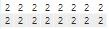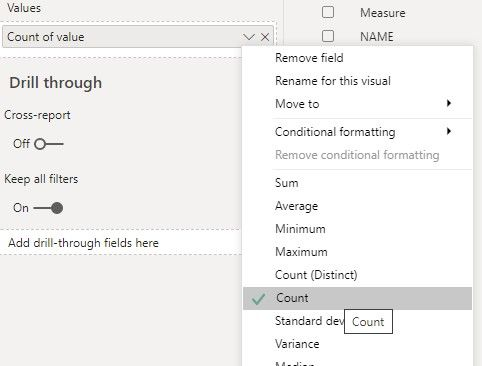Difference when the values are summed.  This is Correct!!!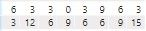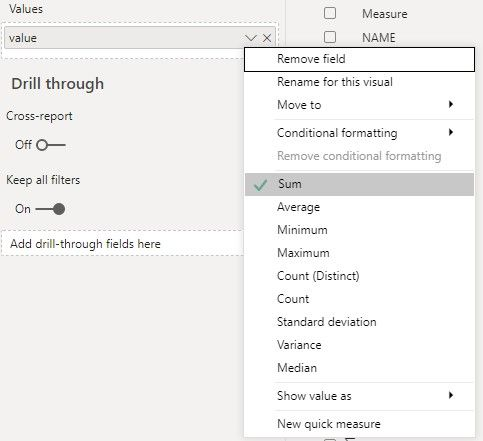Values when showing the Maximum: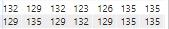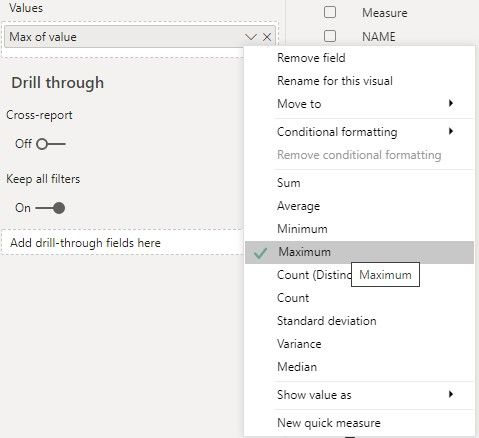Values when showing the Minimum:Values when the data is collapsed.  This is incorrect!  The rows are being summed.  The difference should seen.The data shows correctly in the orginal table.  The new table is the problem.

Hoping someone can help.
5 REPLIES 5Helper V

All the new table scatter plot needs to show is the largest (MAXX) delta at any level in the data hierarchy.

It's not doing that and I'm now I am unsure I am doing things correctly.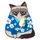Super User

Is it far to assume that your scatter plot is Measure A vs. Measure B?  Or Measure A vs Measure C, etc.Helper V

@littlemojopuppy

Here's an accurate but simplified form of the data and scatter plots involved.
Original vs New Table
Orginal Scatter and Table on the left side,

and New Scatter and Table on the right side.

I want to plot the differences at any level of the data, but the differences are not maintained as the data is collapsed.

The New Table scatter plot should show deltas per Measure along the X-axis:

Y-axis = T0

X-axis = Maxx Delta T0-T1, Maxx Delta T0-T2, Maxx Delta T0-T3Super User

Hi!  Without explaining the mechanics of what you did, can you explain what you're trying to accomplish?  Why did you pivot three measures into a new table?  Because I'm willing to bet there's an easier way to do this.

And can you provide sample data and definition of the measures in question?Helper V

@littlemojopuppy
Sure.

The main reason for the new table is to show all the measures as legend in a Scatter Chart,
and show the results of the measures along the X-axis of the Scatter Chart.

Then I can view all the data along the X-axis as plotted against T in the Y-axis.
Each measure showing as a three distinct groupings in the scatter plot,

In the original table, each measure will show as it's own column.  With only a single measure

getting plotted along the x-axis and showing in the legend at one time.  Not all measure values can be plotted along the X-axis of the scatter chart in the original table.  (I don't know how to get three separate measure into the X-axis and showing in the legend of the scatter chart).

I'll provide a sample set of data.

-Corrected.